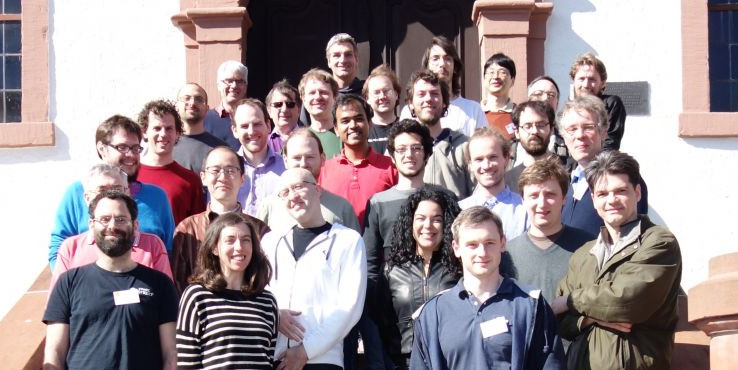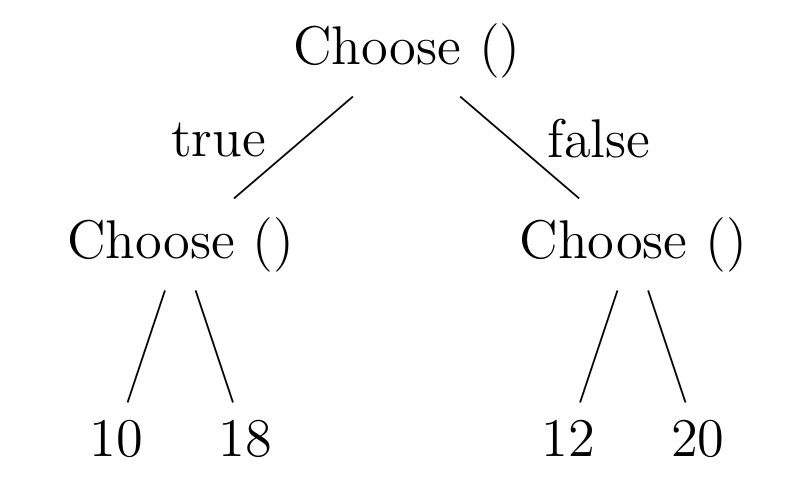# Algebraic effects and handlers

## New language ideas for user-defined side-effects

 Gabriel Scherer Northeastern University gabriel.scherer@gmail.com

## Introduction

### Context

Last March, I went to a Dagstuhl Seminar on

Algebraic Effects and Handlers.I'm talking about what I learned there.

None of the talk is about my own work.

### Algebraic effect and handlers: story

A formal presentation of side-effects.

(Gordon Plotkin and John Power, 2002)

(Gordon Plotkin and Matija Pretnar, 2009)

### Algebraic effect and handlers: implementations

(all in development)

• Eff: Matija Pretnar and Andrej Bauer, 2011-now.
• Links : Daniel Hillerström and Sam Lindley, 2015.
• Frank: Conor McBride, 2007, 2012.
• OCaml+effects: Stephen Dolan, Leo White, KC Sivaramakrishnan, 2016.

### Warmup: ML exceptions

exception Foo of int
let pos n = if n >= 0 then n else raise (Foo n)

let rec map f = function
| [] -> []
| x::xs ->
let a = f x in a :: map f xs

let () =
let pos_input =
try map pos [1; 2; -3; 4] with
| Foo n ->
eprintf "negative input found: %d\n" n;
raise Exit
in List.iter (printf "%d\n") pos_input

### Warmup match .. with exception

Proposed by Christophe Raffali in 2002, but forgotten.

(Better syntax than Benton and Kennedy's “exceptional syntax”)

Implemented by Jeremy Yallop in OCaml 4.02 (Aug 2014), inspired by effect handlers.

match map pos [1; 2; -3; 4] with
| exception (Foo n) ->
eprintf "negative input found: %d\n" n;
raise Exit
| pos_input -> List.iter (printf "%d\n") pos_input

Idea: “value return” vs. “exceptional return”.

### Resumable exceptions?

let pos n = if n >= 0 then n else raise (Foo n)

Resumable exceptions in upstream OCaml. Let's imagine:

try[@resumable] map pos input with
| Foo n k -> continue k (-n)

or

try[@resumable] map pos input with
| Foo n k ->
let n = read_int () in
continue k n

Notice: here we need deep rather than shallow handlers.

Typing? How do we know which type k expects?

### Effect signatures

Effect signature := set of operations

effect choice =
| Choose : bool

effect IO =
| Print : string -> unit

effect int_state =
| Get : int
| Set : int -> unit

effect scheduler =
| Spawn : (unit -> unit) -> unit
| Yield : unit

### Effectful code

Instead of returning a value, one may perform an operation.

let rec in_interval a b : int =
if a = b then a
else
if perform Choose then a
else in_interval (a + 1) b
let incr_twice () : int =
perform (Set ((perform Get) + 1));
perform (Set ((perform Get) + 1));
perform (Print "incremented twice");
perform Get

### Effect handlers: examples

Handle an operation; maybe resume computation.

let a_number =
handle (in_interval 1 10) with
| Choose k -> continue k (Random.bool ())
let state (f : unit -> 'a) : int -> 'a  =
handle f () with
| Get k -> (fun s -> (continue k s) s)
| Set s k -> (fun _ -> (continue k ()) s)
| return v -> (fun _ -> v)

let two = (state incr_twice) 0

### Effect handlers: subsumes exceptions

This subsumes exceptions.

effect exn =
| Foo : int -> empty

let pos n = if n >= 0 then n else perform (Foo n)

handle map pos [1; 2; -3; 4] with
| Foo n k -> (* k cannot be called, no possible argument at type 'empty' *)
| return pos_int -> ...

### Effect handlers: several handlers for an effect

Changing the handler brings expressivity.

let always_true = handler
| Choose k -> continue k true

let one = handle (in_interval 1 10) with always_true
let random = handler
| Choose () k ->
let b = Random.bool () in
continue k b

let random_one = handle (in_interval 1 10) with random
let all_choices = handler
| Choose k -> List.append (continue k true) (continue k false)
| return v -> [v]

let all = handle (in_interval 1 10) with all_choices
(* note: type change *)

### Effect handlers

Change of viewpoint: we are not merely playing with delimited continuations (or resumable exceptions), we are defining side-effects.

(Side-effects implemented through control; “the mother of all monads”)

Claim: this is more structured, makes code easier to understand.

Nota Bene: we can provide different handlers for the same signature.

### Combining handlers

effect backtrack =
| Fail : empty
| Amb : bool -> a

type 'a option =
| None : 'a option
| Some : 'a -> 'a option

let solve = handler
| return v -> Some v
| Fail k -> None
| Amb k ->
(match continue k true with
| Some v -> Some v
| None -> continue k false)

let f () =
if (perform Amb)
then (incr_twice; perform Fail)
else (perform Get)

(handle (state f) 0 with solve) = Some 0
(state (fun () -> handle f () with solve) 0) = Some 2

## User-defined effects, motivation and theory

### Why would user define their own effects?

Okay, Racket has most effects built-in. Delimited control, state, stack marking, concurrency, randomness, dynamic binding, etc.

But combinatorial explosion: you don't support all combinations.

What about mutable state that is rolled back on backtracking?

Also: less runtime, more library.

### (Theory) Monads vs. algebraic effects

Two ways to define algebraic structure: direct construction, or presentation by generators and equations.

Monoid1 := (Nat,1,*)
Monoid2 :=
generators: (a, b)
equations: (b.b = b)
aa
aab
aababaa
abba = aba

Puzzle: what's a direct construction for Monoid2?

It's non-trivial to go from one presentation to the other. For a given application, one may be more convenient.

A Monad m a is any parametrized type m that support two operations

  return : a -> m a
bind : m a -> (a -> m b) -> m b

with some equations that those operations must respect. Examples:

Partial A := Maybe A
Error{E} A := A + E
State{S} A := (S -> A * S)
Concurrent A := List A

A monad is the denotational semantics of an effect.

(It became a programming technique only later.)

### Algebraic effects

Algebraic effects: representation by generators + equations

effect state =
| Get : int
| Set : int -> unit
where (* not actual code *)
(Get; Get) = Get
(Set s; Get) = (Set s; s)
(Set s1; Set s2) = (Set s2)

Equations matter; but languages don't support them yet. Need for usable verification technology.

Handling concurrent effects with Set A or List A.

Constraining the producer vs. quotienting the consumer.

### Relating an algebraic effect and its monad: State

What are the equations on state?

Normal form of a sequence of operations: Get; Set.

Normal form of a term:

let s = perform Get in
let result = ... in
Set (...);
return result
s -> (a * s)

(Want more on this? Read this blog post of Pierre Dagand)

### Aside

Does this equation hold? Does this law hold?

Language support for equational reasoning.

One of the most impactful, overlooked open problems today.

## Going further in theory

### Semantic of imperative programs

Computation trees### Moggi's idea

Computational λ-calculus with effects

⇓ type-transforming translation ⇓

Pure λ-calculus

One effect at a time:

a + get/set        (s → a × s)

A computation tree (above) becomes a values at a richer type (below)

Instead of writing effectful terms in the effectful lambda-calculus, we can write their encoding in the pure lambda-calculus directly.

computation of type a with monadic effects mvalue at type m a.

do
n1 <- get
set (n + 1)
n2 <- get
set (n2 + 1)
n3 <- get
return n3

get >>= \n1 -> set (n1 + 1) >>= \() ->
get >>= \n2 -> set (n2 + 1) >>= \() ->
get >>= \n3 -> return n3

The white lie of purity: do-notation is just as hard to reason about as imperative code.

### Direct style vs. indirect style

Direct style: writing and reasoning on the effectful language.

Indirect sytle: writing and reasoning on the pure encoding.

• Moggi's computational lambda-calculus: direct style.
• CPBV: indirect style.

Both styles are fine.

Direct style semantics models ask an additional, interesting question: what is a semantic characterization of pure programs?

### Free monads vs. effect handlers

Two ways to get different implementations for the same effects.

Free monad over operations S ~ Terms over an effect signature S

perform (op v1 v2 ...)
let x = e1 in e2
return v

Free monad interpreters ~ Effect handlers

Invariant-enforcing representation ~ Algebraic equations

Concurrent A := List A
Concurrent A := Set A

## Going further in practice

### Eff: dynamic effects (1)

type choice = effect
operation decide : unit -> bool
end;;

(* We create an instance of choice to work with. *)
let c = new choice
let choose_one c = handler
| c#decide () k -> k true

with choose_one c handle
let x = (if c#decide () then 10 else 20) in
let y = (if c#decide () then 0 else 5) in
x - y

### Eff: dynamic effects (2)

let choose_all c = handler
| c#decide () k -> k true @ k false
| val x -> [x]

let c = new choice
let d = new choice

let choices =
with choose_one c handle
with choose_all d handle
let x = (if c#decide () then 10 else 20) in
let y = (if d#decide () then 0 else 5) in
x - y
type state s = effect
operation get : unit -> s
operation set : s -> unit
end;;

### Frank: effectful-call-by-value

interface State x = get : x
| put : x -> Unit.

toggle : [State Bool]Bool.
toggle = bind (get!) {x -> semi (put (not x)) x}.

evalState : s -> [State s]a -> a.
evalState   s    v             =  v.
evalState   s    [put s' -> k] =  evalState s' (k Unit).
evalState   s    [get    -> k] =  evalState s  (k s).
interface Send x = send : x -> Unit.
interface Abort = aborting : Zero.

pipe : [Send x]Unit -> [Receive x]y   -> [Abort]y.
pipe         [_]             y         =     y.
pipe        Unit            [_]        =   abort!.
pipe   [send x -> s]   [receive -> r]  =  pipe (s Unit) (r x).

### Encodings

You can also abstract over all monads providing given operations:

class MonadPlus m where
fail :: m a
choose :: m a -> m a -> m a

inInterval : MonadPlus choice => Int -> Int -> choice Int

Monad transformer stacks, Oleg's Eff library.

When do we need new/extended languages? Two debates:

• what are the best designs ?
• do we need them as language features or are libraries enough?

Sometimes library encodings make designs harder to appreciate.